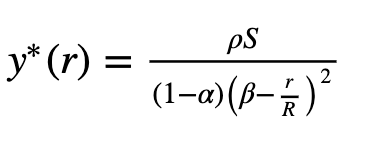# Conviction Voting : Max Ratio/Beta - Deep Dive

### Definition

Max Ratio or β is a value between 0 and 1 which represents the max % of total funds that can be discharged at any given moment. Max Ratio affects the threshold of a proposal. It can be thought of as an “upper ceiling” in asking funds proportional to the total holdings of the Commons.

### Detailed explanation

The max ratio is an important parameter of the threshold calculation. The role of the threshold in the conviction voting algorithm is to determine if a sufficient amount of conviction has accumulated in support of a particular proposal, at which point it becomes funded.

The threshold formula and representation can be seen below, where:

• r is the number of funds requested in the proposal

• R is the total funds in the funding pool

• S is the effective supply which is the total number of tokens used to vote

• ρ is the “Min Effective Supply” a number between 0 and 1 used to weight the effective supply S of governance tokens.### So what do you think the max ratio should be? Do we want a big max ratio so proposals can get a bunch of funds or do we prefer a small max ratio so we can fund many proposals?

2 Likes

We definitely need to look at all the convictions voting parameters together…

A low Max Ratio slow down our spending with CV… but a low half life will counter act that…

I am very excited to see these params modeled so we can really see how all these parameters interact

I’d like to share some tools that will allow us to select beta and min threshold parameters.

We have an interactive graph on how much conviction is required depending on the funds requested. Tweaking beta (β) and min threshold (M) we can see how much conviction is needed.

We can also see how much conviction is gathered during time in this other interactive graph, in which you can interact with the three parameters: half-life, beta and min threshold.

1 Like